American Journal of Biological and Environmental Statistics
Volume 1, Issue 2, September 2015, Pages: 46-50

Realistic Batch Picking Route Optimization Model Using Genetic Algorithms

Vishwa Nath Maurya

Department of Applied Mathematics & Statistics, The University of Fiji, Lautoka, Fiji Islands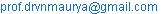Vishwa Nath Maurya. Realistic Batch Picking Route Optimization Model Using Genetic Algorithms. American Journal of Biological and Environmental Statistics. Vol. 1, No. 2, 2015, pp. 46-50.doi: 10.11648/j.ajbes.20150102.11

Received: October 12, 2015; Accepted: May 10, 2016; Published: June 16, 2016

Abstract: Present paper aims to optimize the order batching problem expressed in mathematical language. There, the design and solving steps of order batching problem are based on genetic algorithm. The genetic algorithm in solving process variation method is used to realize the optimization of the model in order to enhance the efficiency of batch picking which increases customer’s satisfaction. Our result explored herein lies that the single order picking equipment limited factor has been extended to multiple sorting equipment in distribution center selection process.

Keyword: Manual Order Picking System, Order Batching, Genetic Algorithm

Contents

1. Introduction

The order picking system of multiple batches and small quantities has received considerable attention of researchers in scenario of rapid growth of e-commerce and the global economy. The artificial picking system occupies a larger proportion in a variety of ways. The optimization of the artificial person picking system has a vital role to enhance the efficiency of batch picking from which customer satisfaction is increased. Optimization and effectiveness of any model in various fields of mathematical and statistical sciences, agricultural and life sciences have received considerable role in research community. Since past few decades, much more noteworthy researchers have confined their attention in this connection. For more details, we refer Gibson and Sharp , Le-Due and De Koste , Maurya and Garg , and Maurya et al. [4,5,6,7,8]. Recently Maurya et al.  confined to explore mathematical modeling and optimization of memorization process to enhance learning using differential equations approach. Here, our keen interest is to design realistic batch picking route problem optimization model using genetic algorithm. The order batching problem expressed in mathematical language is the order needs to be chosen by the right way to carry on the batch. Usually, the model has two objectives to reduce the walking distance or walking time in the selection process. For more details, we refer Gibson and Sharp , and Le-Due and De Koste . Both of the chosen walking time and walking distance have linear correlation relationship. However, chosen walking distance directly determines the walking time in the selection process. This paper is based on the shortest distance as the objective function of the order batching model which propose genetic algorithm of order batching problem. The key reason for application of genetic algorithm is a batch order which has been proved to be NP problem, through accurate calculation method is difficult to obtain the optimal solution of the problem, And the common optimization algorithm has limitations in the optimization of efficiency, it is difficult to be accepted within the scope of the time to find the optimal solutions to the problem, Therefore, Tho Le-Duc  proposed that it is necessary through an intelligent algorithm to solve the target. Distribution center is studied in this paper by artificial picking. Distribution center is labor-intensive. Each picker is picking by operating equipment. There is a number of turnover box on each picking equipment and for each turnover box, there is only one order product. So you can avoid a second sorting process. Picker will directly put turnover box in the export warehouse after he has been completed pick.

2. Proposed Order Picking Model

2.1. Assumptions of the Model

In view of Gilson and Sharp  we make some basic assumptions before mathematical modeling for the convenience of analysis and calculation. A set of our assumptions are as following:

(i) Distribution center storage area is made up of parallel arrangement of shelves. Warehouse-type is dual-zone warehouse, unlike the previous single-area warehouses for scholars. Dual-zone warehouses are more widely available in a large warehouse. The content of this research can be applied to multiple-zone warehouse.

(ii) Number of picking person or picking device for at least one. In previous study, picking people or picking device is set on the amount of a single, in actual operation, there can be multiple selected devices in select situations at the same time, therefore, in our present study, the selected devices are not doing quantity requirements, and that will be more in line with the actual circumstances.

(iii) Ignore the shelf height displacement. In the case of multiple shelves, displacement in vertical direction is not included in the picking path. No matter what the picking strategy we take, the displacement is unable to reduce. It is a fixed value and therefore are not included in the calculation of the chosen path.

(iv) Each order contains at least one kind of product.

(v) The order is not allowed to split into different picking batches, but in the same picking batches, the same order of goods can be assigned to different person to pick.

(vi) The picking orders of each batch are picked by N pickers or picking device at the same time, picking up a batch after batch of the next election.

(vii) Different selection devices with the same capacity limits and load limits.

(viii) The total volume and the total weight of all the goods of each batch picking order cannot exceed the total volume and weight of all selected devices limit in the picking batch.

(ix) The capacity and quality of individual items must not exceed the capacity and quality constraints of the individual picking device, that is to say, picking equipment can picking any goods in the warehouse.

(x) There is no out of stock and emergency insertion order status.

(xi) The picking list goods storage position is known.

(xii) The walking paths in the selection process are improved s-type strategies.

The layout structure is shown in Figure 1.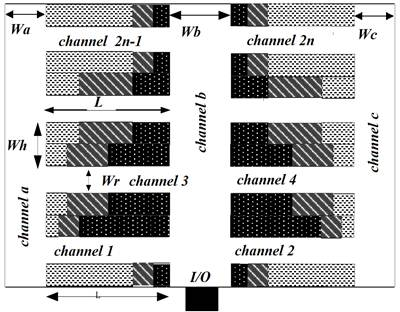Fig.1.Warehouse layout structure.

2.2. Mathematical Formulation of the Model

The mathematical formulation of the proposed order picking model is of the minimization type of objective function as following: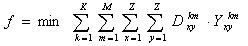(1)

Subject to constraint conditions: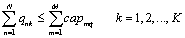(2)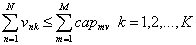(3)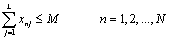(4)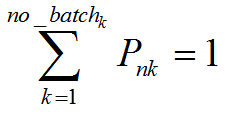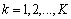(5)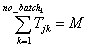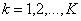(6)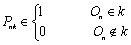(7)(8)(9)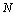is on behalf of total number of orders.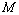is on behalf of the number of picker or picking equipment.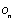is on behalf of orders.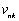is on behalf of the weight of goods in the order n of the batch k.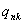is on behalf of the volume of goods in the order n of the batch k.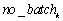is on behalf of the first k picking batch.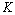is the number of batches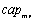is on behalf of the cargo capacity ability of picking equipment m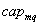is on behalf of the cargo quality ability of picking equipment m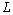is the total number of paths

T is chosen paths j in choose batch k.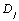is the total distance walked according to the chosen path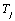.means in the k-th selected batch, walking distance between goods x and goods y.is the decision variables to decide whether the goods y is immediately chose after sorting equipment finished goods x in the first k batch picking.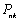is the decision variables to decide whether the order list n in the picking batches k.is the decision variables to decide whether the order list n in the picking path j.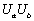is the number of goods a and goods b.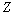is the types of goods.

Here, equation (1) is the objective function of the proposed model. The purpose of the proposed model is to make the total path shortest. Formula (2) represents all the goods volume are not more than the maximum capacity of picking car. Formula (3) represents the quality of all of the goods which does not exceed the maximum loading capacity of picking car. Constraints (2) and (3) are the corresponding model assumes that the eighth rules. Constraint (4) shows that in any one order, the number of picking path can’t exceed the number of picking equipment. Formula (5) represents each order can only be divided in a batch, which is the corresponding model assumes that the fifth rules. Constraints (6) show that in an arbitrary picking batches, the number of chosen path is equal to the number of sorting equipment, The decision variables (7) showed that when order n is included in the batch k, the value is 1, otherwise the value is 0.The decision variables (8) showed that when order n is included in the path j, the value is 1, otherwise the value is 0.Decision variables (9) indicate whether the goods x and y are chosen in a row, if it is in the same batch in the same path next to the selection, the value is 1, otherwise the value is 0.

3. Design of the Genetic Algorithms

In context of designating order batching problem solving steps, we firstly refer Pratik and Russell , Roodbergen and De Koster . We remark here that both of these noteworthy researchers confined their attention to optimize the order batching problem. Here, we propose following procedure consisting of mainly six solving steps using genetic algorithm to design of order batching problem:

Step 1: The Chromosome Coding

In the order batching problem, the order is called a gene, all orders are combined into one chromosome, and each chromosome represents the order batching problem a feasible solution.

Due to the large number of orders, in order to be able to express the solution of the problem by chromosome intuitively, the paper based on the floating-point encoding method. The encoding can be expressed as:

By encoding operating to all orders in the order collection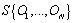, we regard the state of each order in each batch of each vehicle as a gene, and all order status collection form a chromosome. Since the design of the model involves multiple batches and multiple picking equipment, the designed gene should be able to represent order batch and order picking equipment information, or cannot be converted into the corresponding solution space of the chromosome. So for the selection of y value is the form of a-b, where a represents the order batches, and b represents the order picking equipment. For example, a chromosome coding: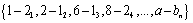The chromosome means: each number position in sequence represents the order number, such as 1-2 in the first place, it represents the order whose coding is 1 in its collection. 2-1 represents the order whose coding is 2.and so on a-b represents the coding order n. Which the number 1-2 represents order in this position is assigned to the first batch of the second picking device; and the number 2-1 represents order in this position is assigned to the second batch of the first picking device. And so on. The middle separator "-" is in order to facilitate the computer recognition of different batches and sorting equipment, through this code, there are multiple picking equipment order batching problem can be mapped to the GA for solving space.

In order batching process, always regard no more than picking equipment selection ability as constraints in batching, and the limit will join in the process of programming. We are not repeating them back.

Step 2: The Population Size

In the design of the parameters chro, if the population is too small, the genetic algorithm is likely to converge to the local optimal solution, not to converge to the optimal solution. The main reason is that population size is too small, will lead to a decrease in the diversity of the population, leading to meaningful searches and the most advantage is lost. On the contrary, if the size of the population is set too large, the calculation needed in each iteration process will become very large, result in the slow, which will affect the practical application value of the algorithm. In this paper, we use the classic genetic algorithm to solve the problem. According to studies conducted by previous scholars, in this paper, chro=20-30, the size of the population to choose the interval values are acceptable.

Step 3: Fitness Function

In the genetic algorithm, the fitness function is used to evaluate the pros and cons of chromosomes in the population, the fitness function and all selection, crossover operation in genetic algorithms have a close relationship and driving force of the GA process. Genetic algorithm carries on the comparison to the fitness of each chromosome in the population, and to determine selection probability based on the ranking, so fitness function f must be positive.

When solving the extreme value of the fitness function, the fitness function can be directly used to model the objective function f (x) to express, also known as the original fitness function. But when the objective function is the opposite, that is to solve the minimum value of the objective function, this method is no longer applicable, Because the optimal objective function value is smaller, when its genetic to the next generation of probability is smaller in accordance with the proportion of genetic. The fitness function need to be appropriately transformed, therefore, make it a standard measure. That is converted to the maximum of the objective function value is optimal. In the case of the objective function for minimization, standard fitness can be defined as: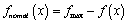Where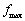is the original fitness function f (x) an upper bound, if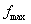belongs to an unknown quantity, can be through the current population of the maximum fitness value as the upper bound of chromosome.

Step 4: Selection of Strategy

In a population, Chromosome according to certain choice probability directly into the next iteration populations, the fitness value of the chromosome is the higher; the probability of being selected is greater. Poor fitness value of chromosome might not have the opportunity to be selected, In the process of selecting, the selection probability for each chromosome is: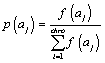The formula is selection probability operator. To calculate selection probability operator according to (3.11) for each chromosome, and then apply the selection operator to select the chromosomes for cross breeding.

Step 5: Crossover Operator

Selecting operation does not produce new chromosomes, Just selecting excellent individuals from the parent generation chromosomes, In order to achieve optimum operation, chromosomal crossover operation must be carried out, Only in this way can produce new excellent individual to implement genetic algorithm optimization process. Through the selection and crossover operator, GA can result in higher average fitness of children group, which is in the optimal direction to evolve. Crossover is the main means of genetic algorithm for optimizing search, by crossover operation to produce the new individual, it greatly accelerate the speed of the search in the process of group evolution.

Select a certain number of chromosomes from the population according to a certain probability, and random group. After the completion of the group, exchange a certain genes of the chromosome from the selected location, the selected location can be one also can be more than one. The probability called the crossover probability. It gives the expected number of participating in the exchange of chromosome, i.e.: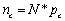Where N is the population size, in this paper, in the process of cross the crossover method used for single point crossover, the specific method is: for each chromosome, randomly generated a floating point number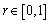. If r<p then the chromosome is selected, repeated selection operation N times, and then all of the selected chromosomes were randomly paired. In chromosome randomly set a new crossing point to crossover after randomly paired, when it crosses, the two chromosomes before or after the point swap part structure. All the finished, forming new groups, such as:

Parent: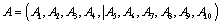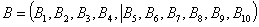Offspring: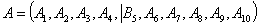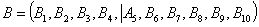Step 6: Mutation Operators

The mutation operation of genetic algorithm is replacing some genes in the chromosome with the other allele of the gene to form a new individual. The important role of the mutation operator is to ensure the diversity of population. And it can make the genetic algorithm find the optimal solution near the current solution.

An important parameter of mutation operation is the mutation probability.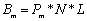is the mutation probability

N is the population size

L is the chromosome length.

In the process of solving the order batching problem, if the mutation probability values of the larger, although able to generate more new chromosome, it may destroy a lot of good search pattern, it will make the genetic algorithm into the stochastic search algorithm; on the contrary if the mutation probability value is too small, then the mutation operation of executive ability is very weak, and the ability of generate new individuals and restrain premature convergence are weak. Combined with genetic algorithm design principles, the range of mutation probability is 0.0001-0.1.

4. Executive Summary

In this paper, an order batching picking route optimization model is established successfully which is to choose walking distance as the optimization goal. The single order picking equipment limited factor is extended to multiple sorting equipment in distribution center selection process. Particularly, single zone simple type warehouse has been extended to double zone warehouse. The model reflects a more realistic batch picking in distribution center. For solving the model, this paper designed the genetic algorithm steps and parameters needed. Population size chro, fitness function f (x), crossover and mutation operators are described and designed.

References

1. Gibson D.R. and Sharp G.P., Order batching procedures, European Journal of Operational Research, Vol. 58(l), pp. 57-67, 2005.
2. Le-Due and De Koste, Travel distance estimation and storage zone optimization in a 2-bloek class-based storage strategy warehouse, Interactional Journal of Production Research, Vol. 43(17), pp. 3561-3581, 2004.
3. Maurya V.N. and Garg Madhu Bala, An alternative approach for determining an optimum assignment schedule in management systems, Acta Ciencia Indica Mathematics, Vol. 32 M, No. 3, pp. 1017-1022, 2006 (Citation No. 015880, Indian Science Abstract, Vol. 43, No. 16, 2007), ISSN: 0970-0455.
4. Maurya V.N., Bathla Rajender Kumar, Maurya Avadhesh Kumar, Arora Diwinder Kaur and Gautam R.A., An alternate efficient sorting algorithm applicable for classification of versatile data, International Journal of Mathematical Modeling and Applied Computing, Academic & Scientific Publishing, New York, USA, Vol.1(1), pp. 1-10, 2013, ISSN: 2332-3744.
5. Maurya V.N., Misra R.B., and Maurya A.K.,Mathematical modeling and optimization of memorization process to enhance learning using differential equations approach, Journal of Cell Science & Theory (JCEST),USA, ISSN 2157-7013, Vol. 4(4), pp 155, 2013.
6. Maurya V.N., Misra R.B., Jaggi Chandra K., Maurya A.K. and Arora D.K., Progressive review and analytical approach for optimal solution of stochastic transportation problems (STP) involving multi choice cost, American Journal of Modeling and Optimization, Science & Education Publishing, USA, Vol. 2(3), pp. 77-83, 2014, ISSN (Print) 2333-1143, ISSN (Online) 2333-1267.
7. Maurya V.N., Reddy Narendra, Maurya Avadhesh Kumar, Datt Nikleshwar, Optimization perspectives and effectiveness of gender in organizational leadership: Scenario of employees satisfaction and organizational goals, IEC University Journal of Engineering, Management and Sciences, India, Vol. 1(1), pp. 15-29, 2014.
8. Maurya Vishwa Nath, Misra Ram Bilas, Jaggi Chandra K., Vashist Swammy, Maurya Avadhesh K., and Shukla Kamlesh Kumar,Two dimensional multiobjective programming problem using graphical approach,American Journal of Biological and Environmental Statistics,Science Publishing Group, USA, Vol. 2(1), 2016.
9. Pratik J. Parikh and Russell D. Meller, Selecting between batch and zone order picking strategies in a distribution center, Transportation Research Part E, Vol.44, pp. 696-719, 2008.
10. Roodbergen K.J. and De Koster R., Routing order pickers in a ware house with a middle aisle. European Journal of Operational Research, Vol.133, pp.32-43, 2001.
11. Tho Le-Duc, Design and control of efficient order picking processes Rotterdam: ErasmusUniversity Rotterdam, 2005.

 Contents 1. 2. 2.1. 2.2. 3. 4.
Article ToolsAbstractPDF(562K)